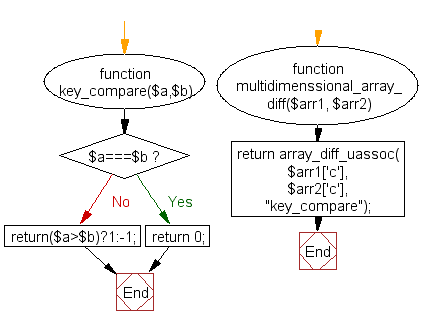﻿ PHP Array Exercise: Difference of two multidimensional arrays - w3resource# PHP Array Exercises : Difference of two multidimensional arrays

## PHP Array: Exercise-57 with Solution

Write a PHP function to compares two multidimensional arrays and returns the difference.

Sample Solution:

PHP Code:

``````<?php
function key_compare(\$a,\$b)
{
if(\$a===\$b)
return 0;
return(\$a>\$b)?1:-1;
}
function multidimenssional_array_diff(\$arr1, \$arr2)
{
return array_diff_uassoc(\$arr1['c'], \$arr2['c'],"key_compare");
}
//multidimenssional arrays
\$color1=array('a'=>'White','b'=>'Red','c'=>array('a'=>'Green','b'=>'Blue','c'=>'Yellow'));
\$color2=array('a'=>'White','b'=>'Red','c'=>array('a'=>'White','b'=>'Red','c'=>'Yellow'));
print_r(multidimenssional_array_diff(\$color1, \$color2));
?>
```
```

Sample Output:

```Array
(
[a] => Green
[b] => Blue
)
```

Flowchart:PHP Code Editor:

What is the difficulty level of this exercise?

﻿

## PHP: Tips of the Day

PHP: Extract numbers from a string

```\$str = 'In My Cart : 11 12 items';
preg_match_all('!\d+!', \$str, \$matches);
print_r(\$matches);
```